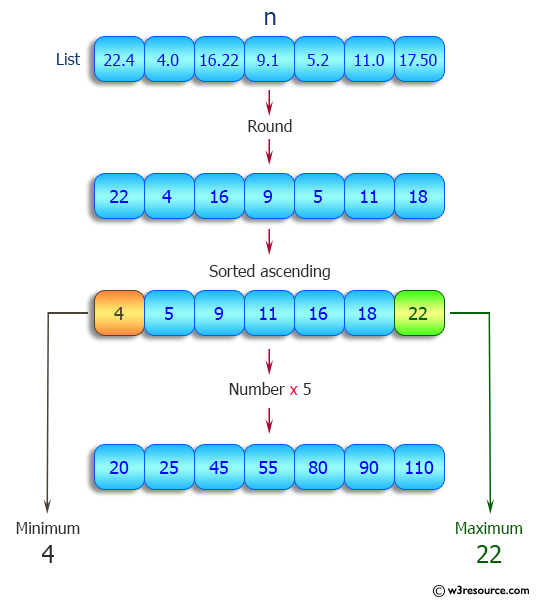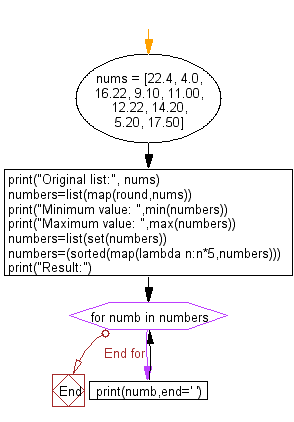﻿ Python: Round the numbers of a given list, print the minimum and maximum numbers and multiply the numbers by 5 - w3resource# Python: Round the numbers of a given list, print the minimum and maximum numbers and multiply the numbers by 5

## Python List: Exercise - 84 with Solution

Write a Python program to round the numbers of a given list, print the minimum and maximum numbers and multiply the numbers by 5. Print the unique numbers in ascending order separated by space.

Sample Solution:

Python Code:

``````nums = [22.4, 4.0, 16.22, 9.10, 11.00, 12.22, 14.20, 5.20, 17.50]
print("Original list:", nums)
numbers=list(map(round,nums))
print("Minimum value: ",min(numbers))
print("Maximum value: ",max(numbers))
numbers=list(set(numbers))
numbers=(sorted(map(lambda n:n*5,numbers)))
print("Result:")
for numb in numbers:
print(numb,end=' ')
```
```

Sample Output:

```Original list: [22.4, 4.0, 16.22, 9.1, 11.0, 12.22, 14.2, 5.2, 17.5]
Minimum value:  4
Maximum value:  22
Result:
20 25 45 55 60 70 80 90 110
```

Pictorial Presentation:Flowchart:## Visualize Python code execution:

The following tool visualize what the computer is doing step-by-step as it executes the said program:

Python Code Editor:

Have another way to solve this solution? Contribute your code (and comments) through Disqus.

What is the difficulty level of this exercise?

Test your Python skills with w3resource's quiz

﻿

## Python: Tips of the Day

```print(2_000_000)
```2000000